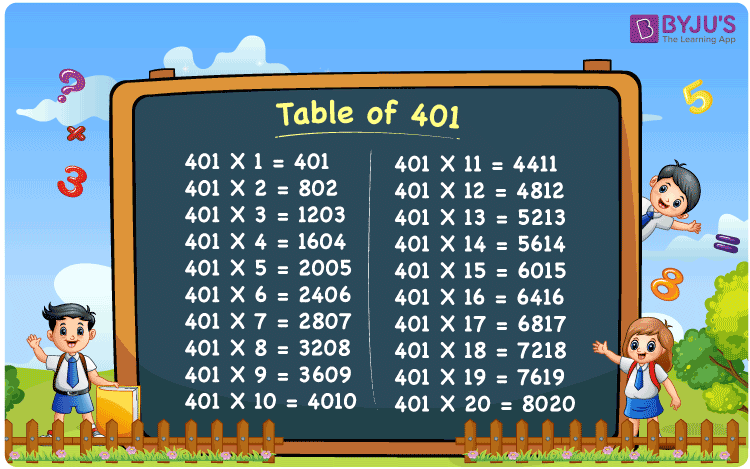Checkout JEE MAINS 2022 Question Paper Analysis : Checkout JEE MAINS 2022 Question Paper Analysis :

# Table of 401

The multiplication table of 401 up to 20 times is presented here, to ease the process of arithmetic calculation. The table of 401 gives the set of values when four hundred and one is multiplied by consecutive natural numbers. 401 times table can also be represented as repeated addition of the original number when multiplied by a number. For example, 401 x 3 = 401 + 401 + 401 = 1203. Multiplication tables help us to solve mathematical problems quickly. Download the PDF of table 401 at BYJU’S for free.

## What is 401 Times Table?The table below shows the repeated addition of the number 401 to get the required multiple.

 401×1 = 401 401 401×2 = 802 401 + 401 = 802 401×3 = 1203 401 + 401 + 401 = 1203 401×4 = 1604 401 + 401 + 401 + 401 = 1604 401×5 = 2005 401 + 401 + 401 + 401 + 401 = 2005 401×6 = 2406 401 + 401 + 401 + 401 + 401 + 401 = 2406 401×7 = 2807 401 + 401 + 401 + 401 + 401 + 401 + 401 = 2807 401×8 = 3208 401 + 401 + 401 + 401 + 401 + 401 + 401 + 401 = 3208 401×9 = 3609 401 + 401 + 401 + 401 + 401 + 401 + 401 + 401 + 401 = 3609 401×10 = 4010 401 + 401 + 401 + 401 + 401 + 401 + 401 + 401 + 401 + 401 = 4010

## Multiplication Table of 401

Here is the table of 401 up to 20 times below.

 401 × 1 = 401 401 × 2 = 802 401 × 3 = 1203 401 × 4 = 1604 401 × 5 = 2005 401 × 6 = 2406 401 × 7 = 2807 401 × 8 = 3208 401 × 9 = 3609 401 × 10 = 4010 401 × 11 = 4411 401 × 12 = 4812 401 × 13 = 5213 401 × 14 = 5614 401 × 15 = 6015 401 × 16 = 6416 401 × 17 = 6817 401 × 18 = 7218 401 × 19 = 7619 401 × 20 = 8020

## Solved Example on Table of 401

Evaluate: 401 x 5 – 2000

Solution: Given,

401 x 5 – 2000

From the table of 401, we have;

401 x 5 = 2005

Therefore,

2005 – 2000 = 5

## Frequently Asked Questions on Table of 401

### What is table of 401 in Maths?

In Maths, the multiplication table of 401 gives the multiples of the original number, such as:

401 x 1 = 401

401 x 2 = 802

401 x 3 = 1203

401 x 4 = 1604

401 x 5 = 2005

### How many times should 401 be added to itself repeatedly to get 3208?

From the table of 401, the product of 401 and 8 is equal to 3208. Thus, 401 should be added eight times to itself to get 3208.

### What is the value of 401 times 20?

401 times 20 is equal to 8020.